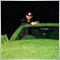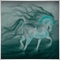34

Hi, I am trying to setup alerts on a line exactly when price touches the line, using bid and ask as per code below giving wrong alert and alerting before the line touches

i want to get alerted when price equals the line, Any help is highly appreciated

here is my code

```static bool     isFirstTick = true;
static datetime m1Time0     = 0;
datetime now                = iTime(_Symbol, 1, 0);

if(m1Time0!=now)
{
if(ObjectFind(0,"My_Line")==0 && counter_up<signals)
{
double Price= ObjectGetDouble(0,"My_Line",OBJPROP_PRICE);
{
m1Time0=now;
isFirstTick=false;
counter_up++;
}
}
}```6217

```if(ObjectFind(0,"My_Line")==0 && counter_up<signals)
```
What is signals?
And why this logicModerator
24865

```         double Price= ObjectGetDouble(0,"My_Line",OBJPROP_PRICE);

All prices on the chart are Bid.

An object's price will be Bid.

You are comparing the price to Ask, not Bid.22749

1. An HLine only has one endpoint, a TLine has two. Don't you want to compare the market to the current value of the line instead of one of its endpoint?
ObjectGetValueByTime
2. You are comparing if the market is above the object. That isn't a touch if you're coming from above. Touch would be bar high and low iare between the line.